• ## 视频质量评价PSNR

千次阅读 2018-05-23 18:16:07
PSNR（Peak Signal to Noise Ratio，峰值信噪比）是最基础的视频质量评价方法。它的取值一般在20-50之间，值越大代表受损图片越接近原图片。PSNR通过对原始图像和失真图像进行像素的逐点对比，计算两幅图像像素点...
        PSNR（Peak Signal to Noise Ratio，峰值信噪比）是最基础的视频质量评价方法。它的取值一般在20-50之间，值越大代表受损图片越接近原图片。PSNR通过对原始图像和失真图像进行像素的逐点对比，计算两幅图像像素点之间的误差，并由这些误差最终确定失真图像的质量评分。该方法由于计算简便、数学意义明确，在图像处理领域中应用最为广泛。

一幅MxN尺寸的图像的PSNR的计算公式如下所示：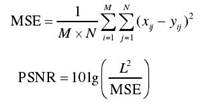其中xij 和yij 分别表示失真图像和原始图像对应像素点的灰度值；
i，j 分别代表图像的行和列；
L 是图像灰度值可到达的动态范围，8位的灰度图像的L=2^8-1=255。
如果已知SSD，MxN尺寸图像的PSNR公式如下所示。

MSE=SSD*1/(M*N)

PSNR=10*lg(255^2/MSE)
例如下图两张1080图片（左边是原图，右边是编码之后的图片，QP为17）的PSNR对比的结果 Y PSNR is 40.632022, U PSNR is 44.596545,V PSNR is 45.759277。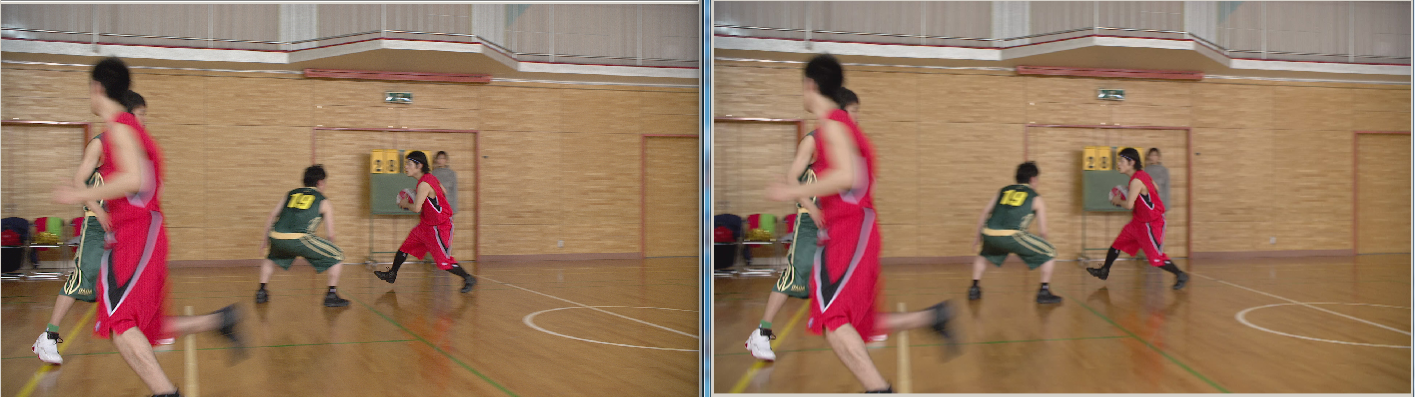PSNR对比测试：1080P视频测试：BasketballDrive_1920x1080_25_250.yuvdp:17 Y PSNR is 40.632022, U PSNR is 44.596545,V PSNR is 45.759277dp:28 Y PSNR is 38.834869, U PSNR is 42.445172,V PSNR is 42.977148dp:32 Y PSNR is 38.144906, U PSNR is 41.265455,V PSNR is 41.705576dp:36 Y PSNR is 37.527187, U PSNR is 40.110563,V PSNR is 40.551498         我们知道量化和反量化过程中，量化步长QP决定量化器的编码压缩率及图像精度。如果QP比较大，则量化值FQ动态范围越小，其相应的编码长度越小，但反量化是损失较多的图像细节，导致PSNR值越小。
代码：
// PSNR_CAL.cpp : 定义控制台应用程序的入口点。
//PSNR (Peak Signal to Noise Ratio)
//峰值信噪比PSNR衡量图像失真或是噪声水平的客观标准。2个图像之间PSNR值越大，则越相似。普遍基准为30dB，30dB以下的图像劣化较为明显。
#include "stdafx.h"
#include <stdlib.h>
#include <stdio.h>
#include <time.h>
#include <math.h>

#define VIDEO_WIDTH			1280
#define VIDEO_HEIGHT		720
#define VIDEO_FRAME_NUM			10 //frame number
//编码解码YUV
#define DEC_YUV_PATH			"E:\\yuv\\\\bs3.yuv"
//原YUV
#define REF_YUV_422			0 //1:reference yuv is 422 format, 0:reference yuv is 420 format
#define VIDEO_SIZE_Y		VIDEO_HEIGHT*VIDEO_WIDTH
#define VIDEO_SIZE_UV		(VIDEO_HEIGHT*VIDEO_WIDTH)>>1
#define VIDEO_SIZE_YUV		(VIDEO_SIZE_Y + VIDEO_SIZE_UV)
#define CONV422	0
#define CAL_PSNR 1

int main()
{
FILE *fp_dec;
FILE *fp_ref;
int i, j, k, comp;

#if CAL_PSNR
unsigned char line_dec;
unsigned char line_ref;
int  idiff;
unsigned long diff_sum;
int width, height;
double psnr_frame;
double psnr_sum[VIDEO_FRAME_NUM];
double psnr_total;

fp_dec = fopen(DEC_YUV_PATH, "rb");
fp_ref = fopen(REF_YUV_PATH, "rb");

if (fp_dec == NULL)
{
return 0;
}

if (fp_ref == NULL)
{
return 0;
}

for (i = 0; i < VIDEO_FRAME_NUM; i++)
{	//Y
for (comp = 0; comp < 3; comp++)
{
diff_sum = 0;
if(comp ==0)
{
width = VIDEO_WIDTH;
height = VIDEO_HEIGHT;
}
else
{
width = VIDEO_WIDTH /2;
height = VIDEO_HEIGHT /2;
}

for (j = 0; j < height; j++)
{

//fwrite(line_ref, 1, width, fp_ref_422);

//if(comp != 0)							//UV
//	fwrite(line_ref, 1, width, fp_ref_422);

for (k = 0; k < width; k++)
{
idiff = (int)(line_dec[k] - line_ref[k]);
diff_sum += idiff*idiff;

//if (k == 0 && j == 5 )
//	printf("stop at %d", k);
}
//if (comp != 0 && REF_YUV_422 == 1) // if 422 format, skip one chroma line
}
psnr_frame = (double)255 * 255 * width* height;
psnr_sum[i][comp] = 10.0 * log10(psnr_frame / (double)diff_sum);
}
printf("frame %d, Y PSNR is %f, Cb PSNR is %f,Cr PSNR is %f \n", i, psnr_sum[i], psnr_sum[i], psnr_sum[i]);
}
psnr_total = 0;
psnr_total = 0;
psnr_total = 0;

for (i = 0; i<VIDEO_FRAME_NUM; i++)
{
psnr_total += psnr_sum[i];
psnr_total += psnr_sum[i];
psnr_total += psnr_sum[i];
}

psnr_total = psnr_total / (VIDEO_FRAME_NUM);
psnr_total = psnr_total / (VIDEO_FRAME_NUM);
psnr_total = psnr_total / (VIDEO_FRAME_NUM);
printf("average PSNR of sequence is:\n Y PSNR is %f, U PSNR is %f,V PSNR is %f\n", psnr_total, psnr_total, psnr_total);

fclose(fp_dec);
fclose(fp_ref);
system("pause");
#endif

#if CONV422
//convert ref 4:2:0 yuv to interleave 4:2:2 yuv
unsigned char Y_plane[VIDEO_SIZE_YUV];
unsigned char U_plane[VIDEO_SIZE_Y / 4];
unsigned char V_plane[VIDEO_SIZE_Y / 4];
unsigned char YUV_plane[2 * VIDEO_SIZE_Y];

unsigned char *pt0;
unsigned char *pt1;
unsigned char *pt2;
unsigned char *pt3;

fp_ref = fopen(REF_YUV_PATH, "rb");
fp_ref_422 = fopen(REF_YUV422_ITLV_PATH, "wb");

if (fp_ref == NULL)
{
return 0;
}

if (fp_ref_422 == NULL)
{
return 0;
}

for (i = 0; i < VIDEO_FRAME_NUM_CONV; i++)
{
fread(U_plane, 1, VIDEO_SIZE_Y / 4, fp_ref);
fread(V_plane, 1, VIDEO_SIZE_Y / 4, fp_ref);

pt0 = Y_plane;
pt1 = U_plane;
pt2 = V_plane;
pt3 = YUV_plane;

for (j = 0; j < VIDEO_HEIGHT / 2; j++)
{
for (k = 0; k < VIDEO_WIDTH / 2; k++)	//the row size of YUV_plane is 2*VIDEO_WIDTH
{
*pt3++ = *pt0++;	//Y0
*pt3++ = *pt1++;	//U0
*pt3++ = *pt0++;	//Y1
*pt3++ = *pt2++;	//V0
}

pt1 = pt1 - VIDEO_WIDTH / 2;
pt2 = pt2 - VIDEO_WIDTH / 2;

for (k = 0; k < VIDEO_WIDTH / 2; k++)	//the row size of YUV_plane is 2*VIDEO_WIDTH
{
*pt3++ = *pt0++;	//Y(WIDTH+0)
*pt3++ = *pt1++;	//U0
*pt3++ = *pt0++;	//Y(WIDTH+1)
*pt3++ = *pt2++;	//V0
}

}

fwrite(YUV_plane, 1, 2* VIDEO_SIZE_Y, fp_ref_422);
}
#endif

}


但是PSNR仅仅计算了图像像素点间的绝对误差，没有考虑像素点间的视觉相关性，更没顾及人类视觉系统的感知特性，所以其评价结果与主观感受往往相差较大(SSIM就是一种典型的与人类视觉系统特性结合的质量评价方法)。
展开全文YUV
• 图像质量的客观评价是指用畸变图像偏离原始图像的误差来衡量畸变图像的质量，目前人们最常用的指标是PSNR（李英明，2011，中国图象图形学报）设 和 分别表示原始图像和待评价图像，且有 PSNR值越大，表示畸变图像...图像信噪比
• 用于计算峰值信噪比，峰值信噪比（Peak Signal to Noise Ratio，PSNR）经常用来评价图像质量
• 我们无论是做实验还是搞别的需求，对图像进行一些处理都可能会让图像的质量受损或者改变内容信息，其改变可以通过图像质量质量评价指标来衡量。 我们提供了两个经常用的指标介绍: 峰值信噪比(Peak Signal-to-Noise ...


文章目录

介绍
我们无论是做实验还是搞别的需求，对图像进行一些处理都可能会让图像的质量受损或者改变内容信息，其改变可以通过图像质量质量评价指标来衡量。 我们提供了两个经常用的指标介绍: 峰值信噪比(Peak Signal-to-Noise Ratio, PSNR) 结构相似度指数(Structural Similarity Index, SSIM)
PSNR
PSNR是“Peak Signal to Noise Ratio”的缩写，即峰值信噪比，是一种评价图像的客观标准，它具有局限性，一般是用于最大值信号和背景噪音之间的一个工程项目。
给定一个大小为 m×n 的原图像 I 与经过处理的图像 K，PSNR 定义为：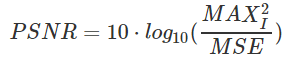其中均方误差（MSE）：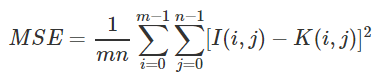MAXI2 为图片可能的最大像素值。如果每个像素都由 8 位二进制来表示，那么就为 255。通常，如果像素值由 B 位二进制来表示，那么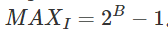SSIM
SSIM(structural similarity index)，结构相似性，是一种衡量两幅图像相似度的指标。SSIM使用的两张图像中，一张为未经压缩的无失真图像，另一张为失真后的图像。
给定两个图像和, 两张图像的结构相似性可按照以下方式求出：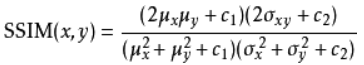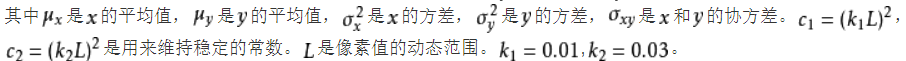结构相似性的范围为0到。当两张图像一模一样时，SSIM的值等于1。
PSNR和SSIM代码实现
参考下一篇博客： 链接https://blog.csdn.net/weixin_43876801/article/details/103146995
参考： 百度百科
展开全文• 为此，以平面图像的客观评价方法PSNR为基础，通过分析人类立体视觉，提出了一种立体图像质量评价方法．该方法分两部分进行立方体图像质量评价，分别是图像质量客观评价与立体感觉客观评价.实验结果表明，该方法的...
• 1. PSNR (Peak Signal-to-Noise Ratio) 峰值信噪比给定一个大小为 $$m×n$$ 的干净图像 $$I$$ 和噪声图像 $$K$$，均方误差 $$(MSE)$$ 定义为：$MSE = \frac{1}{mn}\sum_{i=0}^{m-1}\sum_{j=0}^{n-1}[I(i, j)-K(i,j)... 1. PSNR (Peak Signal-to-Noise Ratio) 峰值信噪比 给定一个大小为 $$m×n$$ 的干净图像 $$I$$ 和噪声图像 $$K$$，均方误差 $$(MSE)$$ 定义为： \[MSE = \frac{1}{mn}\sum_{i=0}^{m-1}\sum_{j=0}^{n-1}[I(i, j)-K(i,j)]^2$
然后 $$PSNR (dB)$$ 就定义为：
$PSNR = 10 \cdot log_{10}(\frac{MAX_I^2}{MSE})$
其中 $$MAX_I^2$$ 为图片可能的最大像素值。如果每个像素都由 8 位二进制来表示，那么就为 255。通常，如果像素值由 $$B$$ 位二进制来表示，那么 $$MAX_I = 2^B-1$$。
一般地，针对 uint8 数据，最大像素值为 255,；针对浮点型数据，最大像素值为 1。
上面是针对灰度图像的计算方法，如果是彩色图像，通常有三种方法来计算。
分别计算 RGB 三个通道的 PSNR，然后取平均值。
计算 RGB 三通道的 MSE ，然后再除以 3 。
将图片转化为 YCbCr 格式，然后只计算 Y 分量也就是亮度分量的 PSNR。
其中，第二和第三种方法比较常见。
# im1 和 im2 都为灰度图像，uint8 类型
# method 1
diff = im1 - im2
mse = np.mean(np.square(diff))
psnr = 10 * np.log10(255 * 255 / mse)
# method 2
psnr = skimage.measure.compare_psnr(im1, im2, 255)
针对超光谱图像，我们需要针对不同波段分别计算 PSNR，然后取平均值，这个指标称为 MPSNR。
2. SSIM (Structural SIMilarity) 结构相似性
$$SSIM$$ 公式基于样本 $$x$$ 和 $$y$$ 之间的三个比较衡量：亮度 (luminance)、对比度 (contrast) 和结构 (structure)。
$l(x,y) = \frac{2\mu_x \mu_y + c_1}{\mu_x^2+ \mu_y^2 + c_1}$
$c(x,y) = \frac{2\sigma_x \sigma_y + c_2}{\sigma_x^2+ \sigma_y^2 + c_2}$
$s(x,y) = \frac{\sigma_{xy} + c_3}{\sigma_x \sigma_y + c_3}$
一般取 $$c_3 = c_2 / 2$$。
$$\mu_x$$ 为 $$x$$ 的均值
$$\mu_y$$ 为 $$y$$ 的均值
$$\sigma_x^2$$ 为 $$x$$ 的方差
$$\sigma_y^2$$ 为 $$y$$ 的方差
$$\sigma_{xy}$$ 为 $$x$$ 和 $$y$$ 的协方差
$$c_1 = (k_1L)^2, c_2 = (k_2L)^2$$ 为两个常数，避免除零
$$L$$ 为像素值的范围，$$2^B-1$$
$$k_1=0.01, k_2=0.03$$ 为默认值
那么
$SSIM(x, y) = [l(x,y)^{\alpha} \cdot c(x,y)^{\beta} \cdot s(x,y)^{\gamma}]$
将 $$\alpha,\beta,\gamma$$ 设为 1，可以得到
$SSIM(x, y) = \frac{(2\mu_x \mu_y + c_1)(2\sigma_{xy}+c_2)}{(\mu_x^2+ \mu_y^2 + c_1)(\sigma_x^2+\sigma_y^2+c_2)}$
每次计算的时候都从图片上取一个 $$N×N$$ 的窗口，然后不断滑动窗口进行计算，最后取平均值作为全局的 SSIM。
# im1 和 im2 都为灰度图像，uint8 类型
ssim = skimage.measure.compare_ssim(im1, im2, data_range=255)
针对超光谱图像，我们需要针对不同波段分别计算 SSIM，然后取平均值，这个指标称为 MSSIM。
获取更多精彩，请关注「seniusen」!展开全文• 基于opencv实现的，测试去噪图像PSNR，图像质量评价
• PSNR（Peak Signal-to-Noise Ratio）峰值信噪比 定义： python代码示例： import numpy as np import math import cv2 original = cv2.imread("edof_preview.jpg") contrast1 = cv2.imread("before....
PSNR（Peak Signal-to-Noise Ratio）峰值信噪比
定义：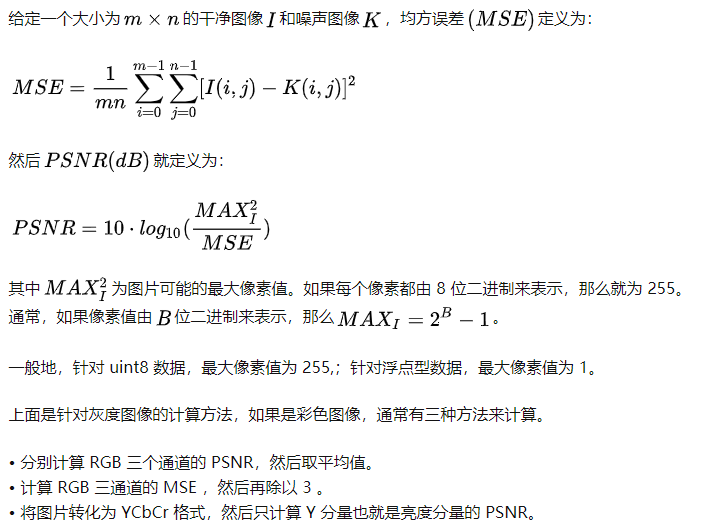python代码示例：
import numpy as np
import math
import cv2

# 如果两幅图分辨率不一样大，可以先resize成一样大
# original=cv2.resize(original,(625,434))

def psnr(img1, img2):
mse = np.mean((img1/255. - img2/255.) ** 2 )
if mse < 1.0e-10:
return 100
PIXEL_MAX = 1
return 20 * math.log10(PIXEL_MAX / math.sqrt(mse))

a=psnr(original,contrast1)

print(a)

参考： https://zhuanlan.zhihu.com/p/50757421
https://blog.csdn.net/qazwsxrx/article/details/104550550/
展开全文python
• 全参考客观视频质量评价方法是指把原始参考视频与失真视频在每一个对应帧中...常用的评价方法包括：均方误差(MSE)、峰值信噪比(PSNR: peak signal to noise ratio)和结构相似性评价(SSIM:structural similarity index
• 评价图像质量 SNR方法 https://blog.csdn.net/itigoitie/article/details/91077307 计算步骤 1.使用待计算SNR图像（记为A）与原始图像（记为B）做相减运算，得到一幅图像（记为C）；我们将B视为A的信息部分，将C视为...matlab 图像处理
• psnr是“Peak Signal to Noise Ratio”的缩写，即峰值信噪比，是一种评价图像的客观标准，它具有局限性，一般是用于最大值信号和背景噪音之间的一个工程项目。 通常在经过影像压缩之后，输出的影像都会在某种程度与...计算机视觉
• 图像质量评价的函数，psnr峰值信噪比，可用于去噪图像和压缩图像的质量评价
• 由于是从Word文档直接复制过来，...图像质量评价指标常用的有PSNR 和 SSIM 一、SSIM，结构相似性 1、定义 SSIM(structural similarity index)，结构相似性，是一种衡量两幅图像相似度的指标。SSIM使用的两张图像中，一.
• f'(i,j)和f(i,j)分别表示的是待评价图像和原始图像，M,N分别表示图像的长与宽. MSE指参数估计值与参数真值之差平方的期望值，在图像处理算法中就是处理后图像像素值与原始像素值之差平方和的均值 二、PSNR（Peak ...
• 计算PSNR的Python代码，网上有下面两种： import cv2 import numpy as np import math def psnr1(img1, img2): mse = np.mean((img1 - img2) ** 2 ) if mse < 1.0e-10: return 100 return 10 * math...
• 图像评价标准SSIMSSIM简介SSIM代码PSNRPSNR简介PSNR代码 SSIM SSIM简介 SSIM也叫做结构相似性，是一种衡量两幅图像相似度的指标，经常用作图像质量评价评价指标包括亮度、对比度和结构比较 简化后的公式如下： ...python 深度学习 SSIM
• PSNR（Peak Signal to Noise Ratio）峰值信噪比，是一种全参考的图像质量评价指标。计算公式如下： 其中，MSE表示当前图像X和参考图像Y的均方误差（MeanSquare Error），H、W分别为图像的高度和宽度；n为每...
• 转自：... OPENCV 有一个example，可以直接用。 ...https://docs.opencv.org/2.4/doc/tutorials/highgui/video-input-psnr-ssim/video-input-psnr-ssim.ht
• matlab有内置函数ssim()、psnr()可以直接调用 ** 计算psnr方法，分为三种： ** 1：计算rgb三通道每个通道的psnr值，再求平均 2：计算rgb三通道每个通道的mse值，再平均，得到psnr。（matlab的内置psnr函数使用此方法...matlab
• Bjøntegaard delta bit rate (BDBR) 表示了在同样的客观质量下，两种方法的码率节省情况(Rate/distortion curves 画一条水平线)  Bjøntegaard delta peak signal-to-noise rate (BD-PSNR)表示了在给定的同等......